# Title 40

## SECTION 53.62

### 53.62 Test procedure: Full wind tunnel test.

§ 53.62 Test procedure: Full wind tunnel test.

(a) Overview. The full wind tunnel test evaluates the effectiveness of the candidate sampler at 2 km/hr and 24 km/hr for aerosols of the size specified in table F-2 of this subpart (under the heading, “Full Wind Tunnel Test”). For each wind speed, a smooth curve is fit to the effectiveness data and corrected for the presence of multiplets in the wind tunnel calibration aerosol. The cutpoint diameter (Dp50) at each wind speed is then determined from the corrected effectiveness curves. The two resultant penetration curves are then each numerically integrated with three idealized ambient particle size distributions to provide six estimates of measured mass concentration. Critical parameters for these idealized distributions are presented in table F-3 of this subpart.

(b) Technical definitions. Effectiveness is the ratio (expressed as a percentage) of the mass concentration of particles of a specific size reaching the sampler filter or filters to the mass concentration of particles of the same size approaching the sampler.

(c) Facilities and equipment required - (1) Wind tunnel. The particle delivery system shall consist of a blower system and a wind tunnel having a test section of sufficiently large cross-sectional area such that the test sampler, or portion thereof, as installed in the test section for testing, blocks no more than 15 percent of the test section area. The wind tunnel blower system must be capable of maintaining uniform wind speeds at the 2 km/hr and 24 km/hr in the test section.

(2) Aerosol generation system. A vibrating orifice aerosol generator shall be used to produce monodisperse solid particles of ammonium fluorescein with equivalent aerodynamic diameters as specified in table F-2 of this subpart. The geometric standard deviation for each particle size generated shall not exceed 1.1 (for primary particles) and the proportion of multiplets (doublets and triplets) in all test particle atmosphere shall not exceed 10 percent of the particle population. The aerodynamic particle diameter, as established by the operating parameters of the vibrating orifice aerosol generator, shall be within the tolerance specified in table F-2 of this subpart.

(3) Particle size verification equipment. The size of the test particles shall be verified during this test by use of a suitable instrument (e.g., scanning electron microscope, optical particle sizer, time-of-flight apparatus). The instrument must be capable of measuring solid and liquid test particles with a size resolution of 0.1 µm or less. The accuracy of the particle size verification technique shall be 0.15 µm or better.

(4) Wind speed measurement. The wind speed in the wind tunnel shall be determined during the tests using an appropriate technique capable of a precision of 2 percent and an accuracy of 5 percent or better (e.g., hot-wire anemometry). For the wind speeds specified in table F-2 of this subpart, the wind speed shall be measured at a minimum of 12 test points in a cross-sectional area of the test section of the wind tunnel. The mean wind speed in the test section must be within ±10 percent of the value specified in table F-2 of this subpart, and the variation at any test point in the test section may not exceed 10 percent of the measured mean.

(5) Aerosol rake. The cross-sectional uniformity of the particle concentration in the sampling zone of the test section shall be established during the tests using an array of isokinetic samplers, referred to as a rake. Not less than five evenly spaced isokinetic samplers shall be used to determine the particle concentration spatial uniformity in the sampling zone. The sampling zone shall be a rectangular area having a horizontal dimension not less than 1.2 times the width of the test sampler at its inlet opening and a vertical dimension not less than 25 centimeters.

(6) Total aerosol isokinetic sampler. After cross-sectional uniformity has been confirmed, a single isokinetic sampler may be used in place of the array of isokinetic samplers for the determination of particle mass concentration used in the calculation of sampling effectiveness of the test sampler in paragraph (d)(5) of this section. In this case, the array of isokinetic samplers must be used to demonstrate particle concentration uniformity prior to the replicate measurements of sampling effectiveness.

(7) Fluorometer. A fluorometer used for quantifying extracted aerosol mass deposits shall be set up, maintained, and calibrated according to the manufacturer's instructions. A series of calibration standards shall be prepared to encompass the minimum and maximum concentrations measured during size-selective tests. Prior to each calibration and measurement, the fluorometer shall be zeroed using an aliquot of the same solvent used for extracting aerosol mass deposits.

(8) Sampler flow rate measurements. All flow rate measurements used to calculate the test atmosphere concentrations and the test results must be accurate to within ±2 percent, referenced to a NIST-traceable primary standard. Any necessary flow rate measurement corrections shall be clearly documented. All flow rate measurements shall be performed and reported in actual volumetric units.

(d) Test procedures - (1) Establish and verify wind speed. (i) Establish a wind speed specified in table F-2 of this subpart.

(ii) Measure the wind speed at a minimum of 12 test points in a cross-sectional area of the test section of the wind tunnel using a device as described in paragraph (c)(4) of this section.

(iii) Verify that the mean wind speed in the test section of the wind tunnel during the tests is within 10 percent of the value specified in table F-2 of this subpart. The wind speed measured at any test point in the test section shall not differ by more than 10 percent from the mean wind speed in the test section.

(2) Generate aerosol. (i) Generate particles of a size specified in table F-2 of this subpart using a vibrating orifice aerosol generator.

(ii) Check for the presence of satellites and adjust the generator as necessary.

(iii) Calculate the physical particle size using the operating parameters of the vibrating orifice aerosol generator and record.

(iv) Determine the particle's aerodynamic diameter from the calculated physical diameter and the known density of the generated particle. The calculated aerodynamic diameter must be within the tolerance specified in table F-2 of this subpart.

(3) Introduce particles into the wind tunnel. Introduce the generated particles into the wind tunnel and allow the particle concentration to stabilize.

(4) Verify the quality of the test aerosol. (i) Extract a representative sample of the aerosol from the sampling test zone and measure the size distribution of the collected particles using an appropriate sizing technique. If the measurement technique does not provide a direct measure of aerodynamic diameter, the geometric mean aerodynamic diameter of the challenge aerosol must be calculated using the known density of the particle and the measured mean physical diameter. The determined geometric mean aerodynamic diameter of the test aerosol must be within 0.15 µm of the aerodynamic diameter calculated from the operating parameters of the vibrating orifice aerosol generator. The geometric standard deviation of the primary particles must not exceed 1.1.

(ii) Determine the population of multiplets in the collected sample. The multiplet population of the particle test atmosphere must not exceed 10 percent of the total particle population.

(5) Aerosol uniformity and concentration measurement. (i) Install an array of five or more evenly spaced isokinetic samplers in the sampling zone (paragraph (c)(5) of this section). Collect particles on appropriate filters over a time period such that the relative error of the measured particle concentration is less than 5.0 percent.

(ii) Determine the quantity of material collected with each isokinetic sampler in the array using a calibrated fluorometer. Calculate and record the mass concentration for each isokinetic sampler as:

Equation 7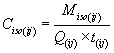where: i = replicate number; j = isokinetic sampler number; Miso = mass of material collected with the isokinetic sampler; Q = isokinetic sampler volumetric flow rate; and t = sampling time.

(iii) Calculate and record the mean mass concentration as:

Equation 8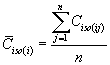where: i = replicate number; j = isokinetic sampler number; and n = total number of isokinetic samplers.

(iv) Precision calculation. (A) Calculate the coefficient of variation of the mass concentration measurements as:

Equation 9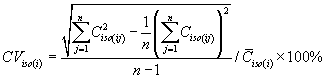where: i = replicate number; j = isokinetic sampler number; and n = total number of isokinetic samplers.

(B) If the value of CViso(i) for any replicate exceeds 10 percent, the particle concentration uniformity is unacceptable and step 5 must be repeated. If adjustment of the vibrating orifice aerosol generator or changes in the particle delivery system are necessary to achieve uniformity, steps 1 through 5 must be repeated. When an acceptable aerosol spatial uniformity is achieved, remove the array of isokinetic samplers from the wind tunnel.

(6) Alternative measure of wind tunnel total concentration. If a single isokinetic sampler is used to determine the mean aerosol concentration in the wind tunnel, install the sampler in the wind tunnel with the sampler nozzle centered in the sampling zone (paragraph (c)(6) of this section).

(i) Collect particles on an appropriate filter over a time period such that the relative error of the measured concentration is less than 5.0 percent.

(ii) Determine the quantity of material collected with the isokinetic sampler using a calibrated fluorometer.

(iii) Calculate and record the mass concentration as Ciso(i) as in paragraph (d)(5)(ii) of this section.

(iv) Remove the isokinetic sampler from the wind tunnel.

(7) Measure the aerosol with the candidate sampler. (i) Install the test sampler (or portion thereof) in the wind tunnel with the sampler inlet opening centered in the sampling zone. To meet the maximum blockage limit of paragraph (c)(1) of this section or for convenience, part of the test sampler may be positioned external to the wind tunnel provided that neither the geometry of the sampler nor the length of any connecting tube or pipe is altered. Collect particles for a time period such that the relative error of the measured concentration is less than 5.0 percent.

(ii) Remove the test sampler from the wind tunnel.

(iii) Determine the quantity of material collected with the test sampler using a calibrated fluorometer. Calculate and record the mass concentration for each replicate as:

Equation 10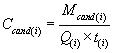where: i = replicate number; Mcand = mass of material collected with the candidate sampler; Q = candidate sampler volumetric flow rate; and t = sampling time.

(iv)(A) Calculate and record the sampling effectiveness of the candidate sampler as:

Equation 11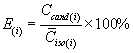where: i = replicate number.

(B) If a single isokinetic sampler is used for the determination of particle mass concentration, replace Ciso(i) with Ciso.

(8) Replicate measurements and calculation of mean sampling effectiveness. (i) Repeat steps in paragraphs (d)(5) through (d)(7) of this section, as appropriate, to obtain a minimum of three valid replicate measurements of sampling effectiveness.

(ii) Calculate and record the average sampling effectiveness of the test sampler for the particle size as:

Equation 12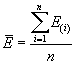where: i = replicate number; and n = number of replicates.

(iii) Sampling effectiveness precision. (A) Calculate and record the coefficient of variation for the replicate sampling effectiveness measurements of the test sampler as:

Equation 13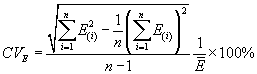where: i = replicate number, and n = number of replicates.

(B) If the value of CVE exceeds 10 percent, the test run (steps in paragraphs (d)(2) through (d)(8) of this section) must be repeated until an acceptable value is obtained.

(9) Repeat steps in paragraphs (d)(2) through (d)(8) of this section until the sampling effectiveness has been measured for all particle sizes specified in table F-2 of this subpart.

(10) Repeat steps in paragraphs (d)(1) through (d)(9) of this section until tests have been successfully conducted for both wind speeds of 2 km/hr and 24 km/hr.

(e) Calculations - (1) Graphical treatment of effectiveness data. For each wind speed given in table F-2 of this subpart, plot the particle average sampling effectiveness of the candidate sampler as a function of aerodynamic particle diameter (Dae) on semi-logarithmic graph paper where the aerodynamic particle diameter is the particle size established by the parameters of the VOAG in conjunction with the known particle density. Construct a best-fit, smooth curve through the data by extrapolating the sampling effectiveness curve through 100 percent at an aerodynamic particle size of 0.5 µm and 0 percent at an aerodynamic particle size of 10 µm. Correction for the presence of multiplets shall be performed using the techniques presented by Marple, et al (1987). This multiplet-corrected effectiveness curve shall be used for all remaining calculations in this paragraph (e).

(2) Cutpoint determination. For each wind speed determine the sampler Dp50 cutpoint defined as the aerodynamic particle size corresponding to 50 percent effectiveness from the multiplet corrected smooth curve.

(3) Expected mass concentration calculation. For each wind speed, calculate the estimated mass concentration measurement for the test sampler under each particle size distribution (Tables F-4, F-5, and F-6 of this subpart) and compare it to the mass concentration predicted for the reference sampler as follows:

(i) Determine the value of corrected effectiveness using the best-fit, multiplet-corrected curve at each of the particle sizes specified in the first column of table F-4 of this subpart. Record each corrected effectiveness value as a decimal between 0 and 1 in column 2 of table F-4 of this subpart.

(ii) Calculate the interval estimated mass concentration measurement by multiplying the values of corrected effectiveness in column 2 by the interval mass concentration values in column 3 and enter the products in column 4 of table F-4 of this subpart.

(iii) Calculate the estimated mass concentration measurement by summing the values in column 4 and entering the total as the estimated mass concentration measurement for the test sampler at the bottom of column 4 of table F-4 of this subpart.

(iv) Calculate the estimated mass concentration ratio between the candidate method and the reference method as:

Equation 14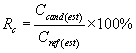where: Ccand(est) = estimated mass concentration measurement for the test sampler, µg/m 3; and Cref(est) = estimated mass concentration measurement for the reference sampler, µg/m 3 (calculated for the reference sampler and specified at the bottom of column 7 of table F-4 of this subpart).

(v) Repeat steps in paragraphs (e) (1) through (e)(3) of this section for tables F-5 and F-6 of this subpart.

(f) Evaluation of test results. The candidate method passes the wind tunnel effectiveness test if the Rc value for each wind speed meets the specification in table F-1 of this subpart for each of the three particle size distributions.